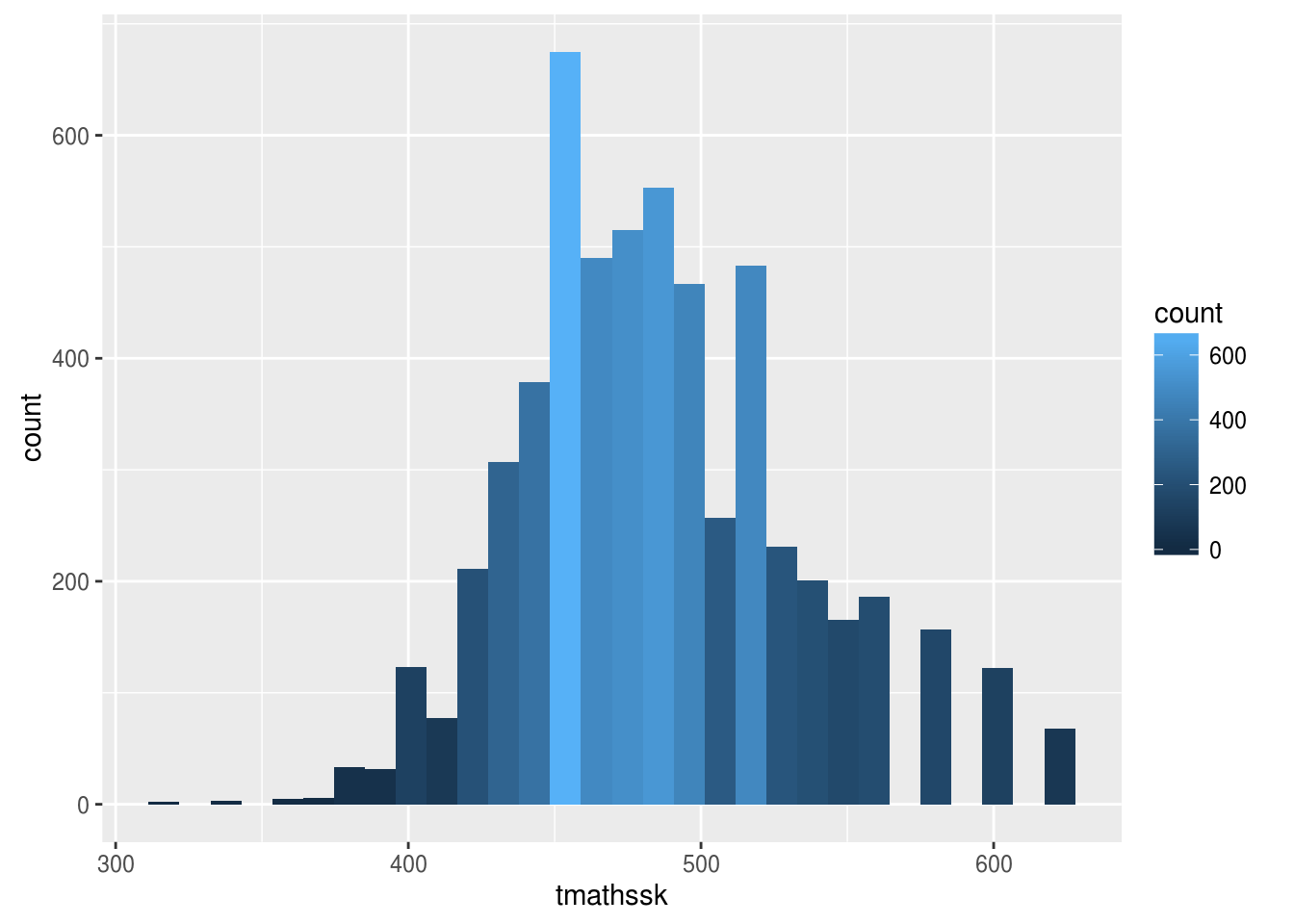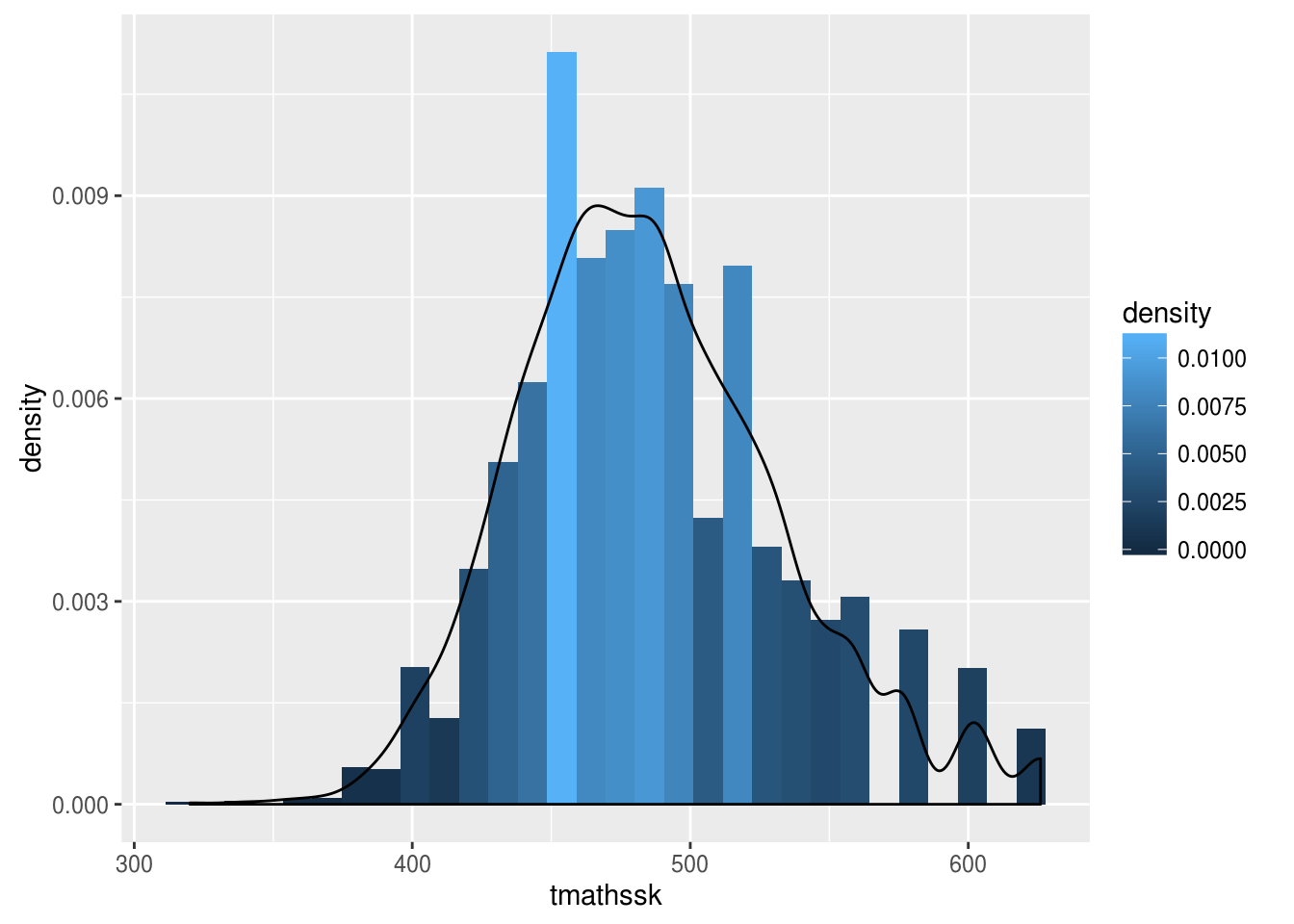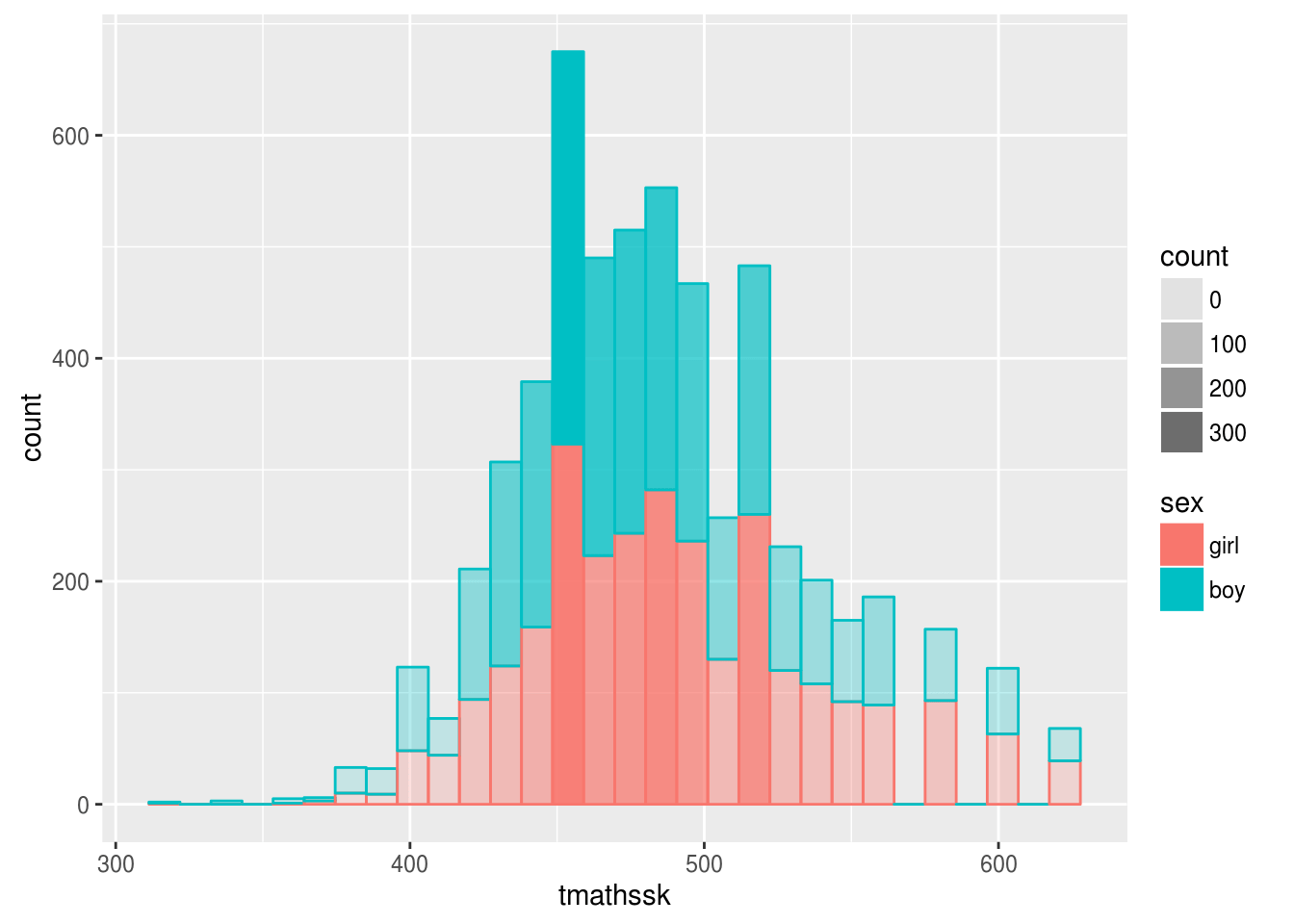# Histograms and Colors with ggplot2

In this post, we will look at how ggplot2 is able to create variables for the purpose of providing aesthetic information for a histogram. Specifically, wewill look at how ggplot2 calculates the bin sizes and then assigns colors to each bin depending on the count or density of that particular bin.

To do this we will use dataset called “Star” from the “Edat” package. From the dataset, we will look at total math score and make several different histograms. Below is the initial code you need to begin.

``library(ggplot2);library(Ecdat)``
``data(Star)``

We will now create our initial histogram. What is new in the code below is the “..count..” for the “fill” argument. This information tells are to fill the bins based on their count or the number of data points that fall in this bin. By doing this, we get a gradation of colors with darker colors indicating more data points and lighter colors indicating fewer data points. The code is as follows.

``ggplot(Star, aes(tmathssk, fill=..count..))+geom_histogram()``
``

As you can see, we have a nice histogram that uses color to indicate how common data in a specific bin is. We can also make a histogram that has a line that indicates the density of the data using the kernel function. This is similar to adding a LOESS line on a plot. The code is below.

``ggplot(Star, aes(tmathssk)) + geom_histogram(aes(y=..density.., fill=..density..))+geom_density()``
``

The code is mostly the same but we moved the “fill” argument inside “geom_histogram” function and added a second “aes” function. We also included a y argument inside the second “aes” function. Instead of using the “..count..” information we used “..density..” as this is needed to create the line. Lastly, we added the “geom_density” function.

The chart below uses the “alpha” argument to add transparency to the histogram. This allows us to communicate additional information. In the histogram below we can see visual information about gender and the how common a particular gender and bin are in the data.

``ggplot(Star, aes(tmathssk, col=sex, fill=sex, alpha=..count..))+geom_histogram()``
``

Conclusion

What we have learned in this post is some of the basic features of ggplot2 for creating various histograms. Through the use of colors, a researcher is able to display useful information in an interesting way.